# The sum of the two largest eigenvalues (Solved)

 Importance: Medium ✭✭
 Author(s): Gernert, Dieter
 Subject: Graph Theory » Algebraic Graph Theory
 Keywords: eigenvalues spectrum
 Recomm. for undergrads: no
 Posted by: mdevos on: June 6th, 2007
 Solved by: Vladimir Nikiforov, Linear Combinations of Graph Eigenvalues, Electronic Journal of Linear Algebra 15 pp 329-336
Problem   Letbe a graph onvertices and let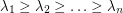be the eigenvalues of. Is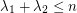?

This property does hold for all regular graphs. Ifis-regular, then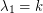. Further, if we let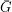denote the complement ofand letdenote its eigenvalues, then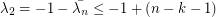(the second inequality here follows from the observation thatis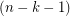-regular).

## Bibliography

Open Problems in Spectral Graph Theory (a list maintained by Dragan Stevanović).

* indicates original appearance(s) of problem.

### Solved!

About 5 months ago, I was shown a counterexample to this conjecture by Bojan Mohar (I believe in joint work with two of his grad. students - Javad Ebrahimi and Azhvan Sheikh). However, thanks to Gordon Royle, I have just learned that this problem was resolved earlier by Vladimir Nikiforov. See Linear combinations of graph eigenvalues.

### Is this really solved?

I'm confused how the paper's result (which you have posted) solves this question. I didn't read the paper, but the abstract only gave a looser bound that the sum of the first two eigenvalues is <= 2/sqrt(3) * n (and not just n).

### a negative solution

The paper shows that the sum of the first two eigenvalues exceeds 1.125n - 25, so exceeds n when n is sufficiently large. Thus Nikiforov has given a negative solution to the problem. (Note: I have not checked the claims of the paper thoroughly.)

### Is this really solved?

Yes. Nikiforov's inequality says that for all n >= 21 the maximum of the sum of the two largest eigenvalues of a graph on n vertices is at least f(n):=n(29+sqrt(329))/42-25. Notice that for n >= 204, f(n)-n is positive. Thus, for all n >= 204 there is a graph with n vertices and with the sum of the two largest eigenvalues greater than n.

Take a look at http://garyedavis.wordpress.com/ for an example and 3D picture of a graph on 40 vertices, with 770 edges for which the sum of the two largest eigenvalues is > 40.

I don't know if anyone knows the least n for which there is a graph with n vetices such that the sum of the two largest eigenvalues is greater than n.

Regards,

Gary Davis

## Comment viewing options

Select your preferred way to display the comments and click "Save settings" to activate your changes.